## Uniform and Discrete Distribution using the TiNspire CX

Given a Discrete Probability Distribution such as the one shown below make sure that the sum of the given probabilities is 100% or 1 . If you own a TiNspire CX you can enter the distribution in the top two boxes of the Statistics Made Easy App available at www.TiNspireApps.com .

Once done you get the expected value , variance : step by step.
Optional is the value of N which allows to compute P(X>N) , P(X<N) etc

For a uniform distribution , you enter the given interval [a,b] and the value c which lies on [a,b] . You will then find P(X<c) and P(X>c)

Posted on Categories discrete math, distribution, statistics

## Confidence Intervals and Margin of Error using the TiNSpire CX

To find Confidence Intervals of Proportions using Statistics Made Easy at  https://www.tinspireapps.com/?a=SPME use option 7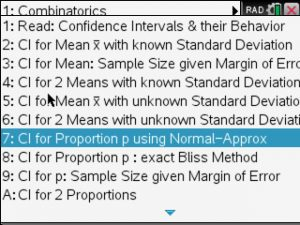Next, the given proportion po , sample size n and the confidence level alpha as shown below: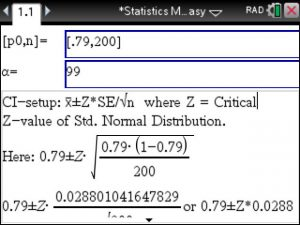Automatically, the correct setup and solution shows in the bottom window.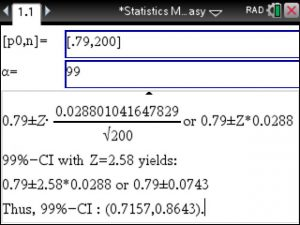To also find the sample size given the Margin of Error use option 9: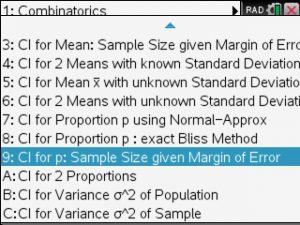you will enter the given Margin of Error, proportion and the confidence level Alpha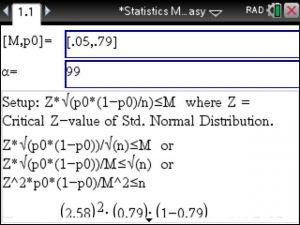Again, the correct setup is shown and the sample size is computed step by step, here we get n>=441.7187Posted on Categories statistics

## SOLVED: Normal Distribution & Sampling Distribution (TiNspire)

Below is a typical Normal Distribution problem that will be solved further below using the Statistics Made Easy app .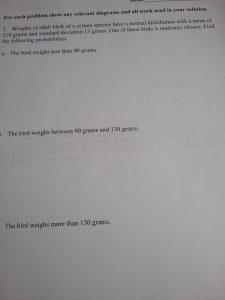Using the Normal Distribution feature in the Statistics Made Easy app , we enter the given mean and standard deviation in the top box use 0 and 19 for A and B to 0.0324 or 3.24% .For question 2 we use 90 and 130 instead in the 2. box to 85.83%For the 3. question we use 130 as A and some large B such as 500 (or 1000) to get 0.1092 or 10.92% as the answer.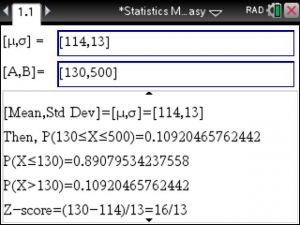For Sampling Distribution we use the Central Limit Theorem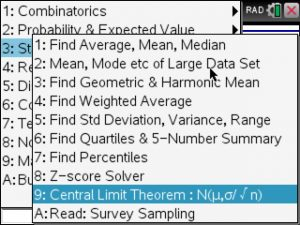and enter the known mean, standard deviation and sample size to get the sampling distribution

N(mean, std dev/square root(n) ). Here we get 114 and 1.3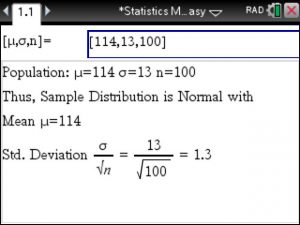Since the sampling distribution is a normal distribution , we go to the previous and only change the standard deviation to 1.3 instead of 13. Below, we type in the given 110 and 116 to get 93.6986%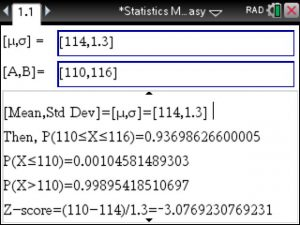That was not too difficult at all! It was easy..

Posted on Categories statistics, tinspirecx

## Lower & Upper Inner (Outer) Fence , IQR , 5 Number Summary (Tinspire CX)

If your Stats teacher is asking you to compute things such as:

• Lower & Upper Inner (Outer) Fence
• IQR
• 5 Number Summary

and you have a Tinspire CX CAS then head over to Statistics Made Easy which actually makes Statistics a lot easier: Finding Mean, Median, Mode, Standard Deviation, Variance, Fences, IQR, Statistical Tests, Distributions, Confidence Intervals and a whole lot more …

## Correlation Coefficient and Coefficient of Determination using the TiNSpire – Step by Step

Linear Regression you just type in the given x and y values. Next the linear regression is computed for you along with the  Correlation Coefficient and the Coefficient of Determination.

That simple.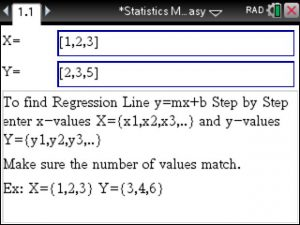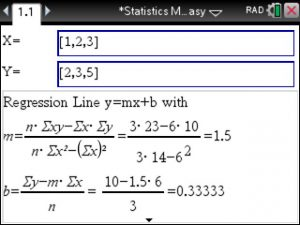Posted on Categories statistics, tinspirecx

## ▷Statistics Made Easy User Review: It really helped me understand through a different point of view.

I love the Statistics features, it really helped me understand through a different point of view.

I’ve always dreamed of an application like you have created!

Thank you for following your dreams and making these TiNspire applications.

Posted on Categories statistics, testimonial

## Mean, Mode, Median, Standard Deviation of LARGE data sets using the TiNspire CX

Say you have 10 thirteens, 10 twos and 500 threes and need to find

Mean, Mode, Median, Standard Deviation, Variance etc. To avoid looooots of typing you can

now enter such LARGE data using the TiNspire’s Statistics Made Easy app at www.TiNspireApps.com

Enter data as simple as : [10,10,500]   and [13,2,3]

Voila! That simple!

We love your suggestions, keep them coming. This feature was suggested by user Steve L. GREAT suggestion!

Posted on Categories statistics

## Confidence Interval for Proportions using the exact Bliss Method on the TiNspire

When finding Confidence Intervals for Proportions we typically involve the Normal Distribution to create an confidence Interval estimate.

However, this method requires the conditions np<5 and n(1-p)<5 to be fulfilled.

It is not uncommon to have a large sample size n available which likely disables this method.

This is where the Bliss Method comes in to find the exact Confidence Interval on the TiNspire.

Using Statistics Made Easy at www.TiNspireApps.com  use menu option 6 then 8 to perform the Bliss method step by step.

Posted on Categories statistics

## Your apps have really saved the day and helped me understand Chemistry at a deeper level!

Customer Testimonial:

Your apps have really saved the day, when i was in my chemistry class it really helped me understand it at a deeper level.

I got a B in that Class i never thought in my life i would get a B in Chemistry.

These apps really helped me learn Balancing chemical equations, Helped me learn the periodic table, and helped me learn stoichiometry.

I have switched to computer science and will only need 1 more chemistry class but i was glad i had it.

It really saved the day when it came to test time.

At 49.95 it is a steal.

When i got the Business and Statistics made easy it really helps me to visualize it

## Hypotheses Tests using the TINspire in Statistics Made Easy (www.TiNspireApps.com)

This will show how easily Hypothesis Test at a given Significance Level can be performed – Step by Step –
using the TiNspire’s Statistics Made Easy at www.TiNspireApps.com

Here is problem that calls for a 2 sided Ztest with given Std Deviation: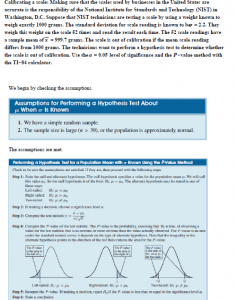Using the Z-test, select the 2 sided test (not equal symbol) at the 5 % level and

entering the 4 values pertaining to the test as shown below: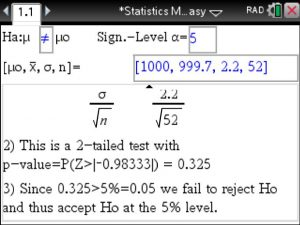We immediately see the computed p-value and the drawn conclusion (here we fail to reject Ho as the p value larger than 5%)Besides Significance Tests the Statistics Made Easy is really useful when
performing Confidence Intervals and also Non Parametric Test available at
www.TiNspireApps.com

Posted on Categories hypothesis test, statistics, steps